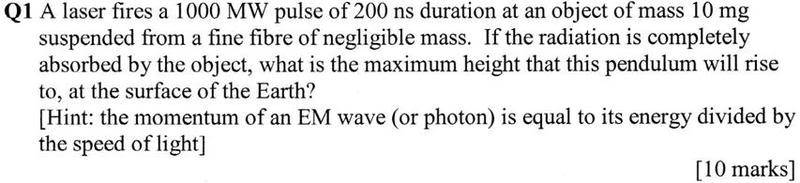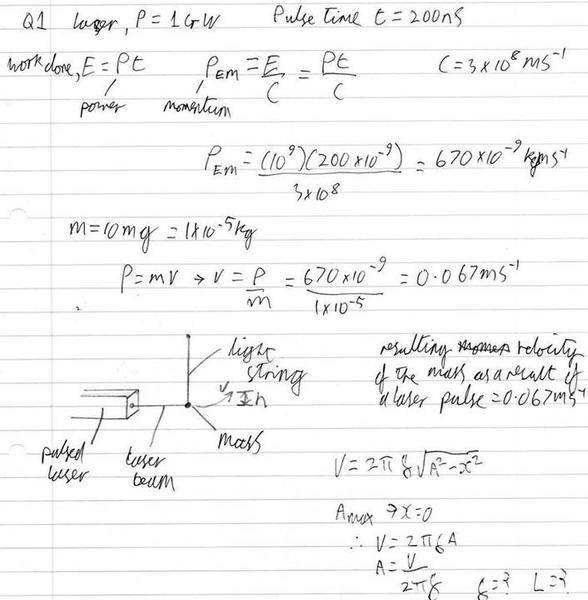# SHM problem, involves a laser.

I am struggling with the following question:My attempt so far is:(in the diagram h is supposed to be A)
(i have also assumed the pulse time is negligible, so the mass starts at the resultant velocity)

The set of homework questions this has come from is supposed to be related to Maxwell's Equations but this one seems to have become a mechanics problem. After reading the question i first thought that the laser would be pushing the mass directly upward against gravity, but after calculating the resultant velocity and re-reading the question my best guess is that it's supposed to be pushing the mass horizontally while it is suspended from a string.

This would be SHM i thought and the amplitude would be the height increase. My problem now is the problem doesn't give the length of the sting and i can't find a way to calculate f or A without using l.

Any help much appreciated.

Dick
Homework Helper
The question doesn't ask for the amplitude of the oscillation. It's only asks for the maximum height. Use conservation of mechanical energy.

OK, i was barking up the wrong tree, after equating E(p) and E(k) i got h=v2/(2g), after plugging in the values i got about 2.3x10^-4m. This seems quite small for a 200J energy input but i guess it's reasonable as the force light exerts is minuscule?

Dick